This section deals with the variations of the correlation coefficient and nonparametric statistical procedures. Comparisons will be made between various parametric and nonparametric statistics.  The correlation is a parametric statistic but is used here as a starting point or comparison for nonparametric statistics.

Nonparametric Statistics

The first set data to be used for the examples that follows:

Chitest1.sav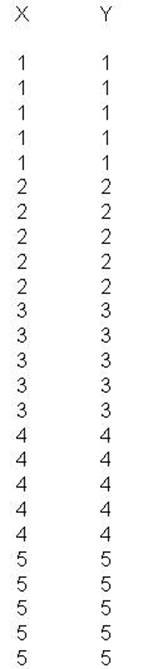The following clicks will produce the resulting Syntax File

Click on Analyze
Click on Correlate
Click on Bivariate
Select both X and Y Variables
Click on right delta button
Click on Options
Select Means and Standard Deviations
Click Continue
Click Paste
Type in "Get file = 'e:/dape/chitest1.sav'."
Click Run
Click All

The above clicks will produce the following syntax file and output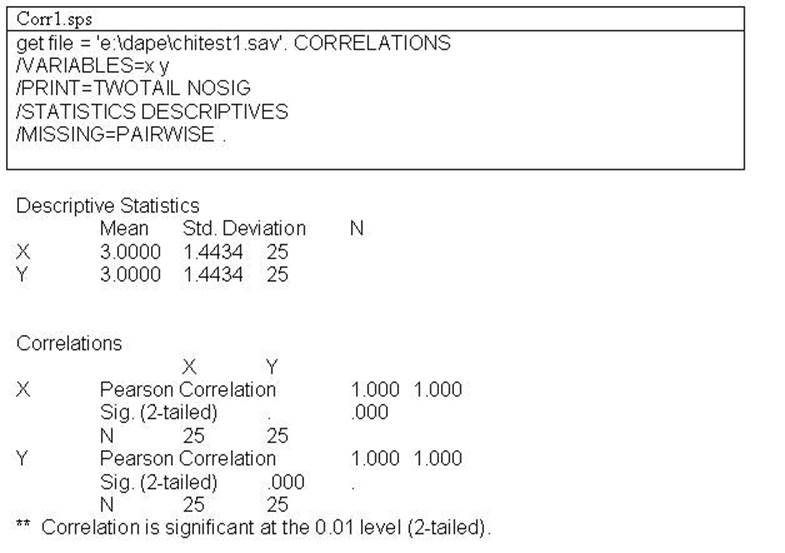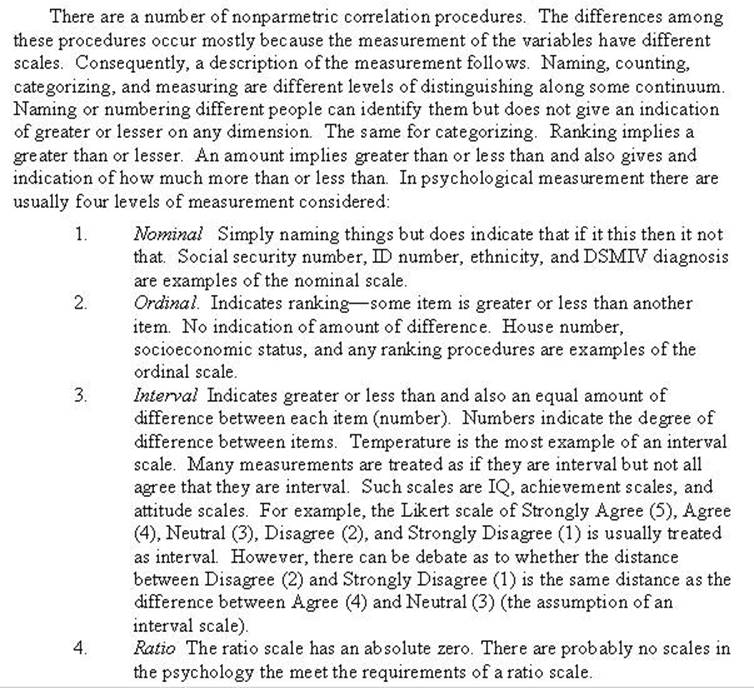Two types of Nonparmetric Procedures (Correlational & Test group differences)

A. Nonparametric Correlation Procedures

Nonparametric procedures allows one to correlate variables when their scales are nominal and ordinal. Consequently, nonparametric procedures are used when the scale of measurement of the data are only at the level of nominal or ordinal. The output from the various programs indicate level of measurement. The next set of clicks produces and number [ Correlation Nonparametric ]

(assumes file "chitest1.sav" is open ).

Click Analyze
Click Descriptive Statistics
Click Crosstabs
Select the X variable Click delta button
Select the Y variable Click delta button
Click Statistics
Select all desired statistics by clicking in the corresponding box
Click Continue
Click Paste
Click Run
Click All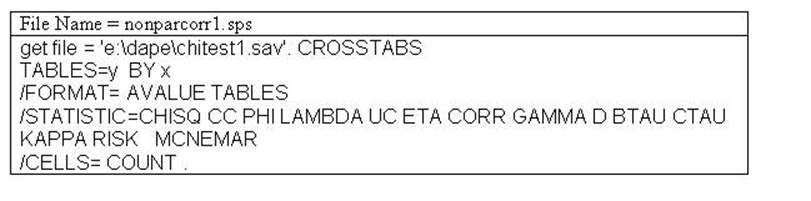The
syntax file (nonparcorr1.sps) program with the data labeled "chitest1.sav' data produces the following output: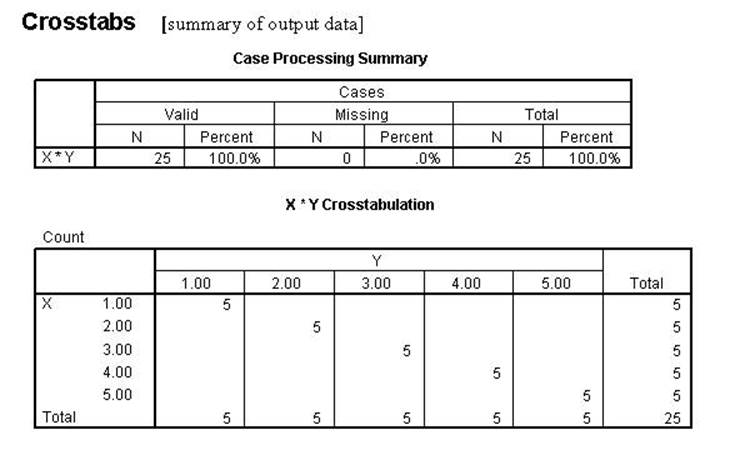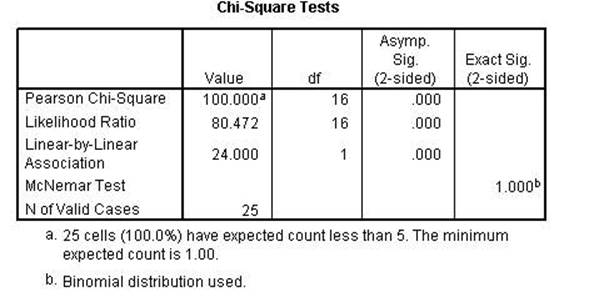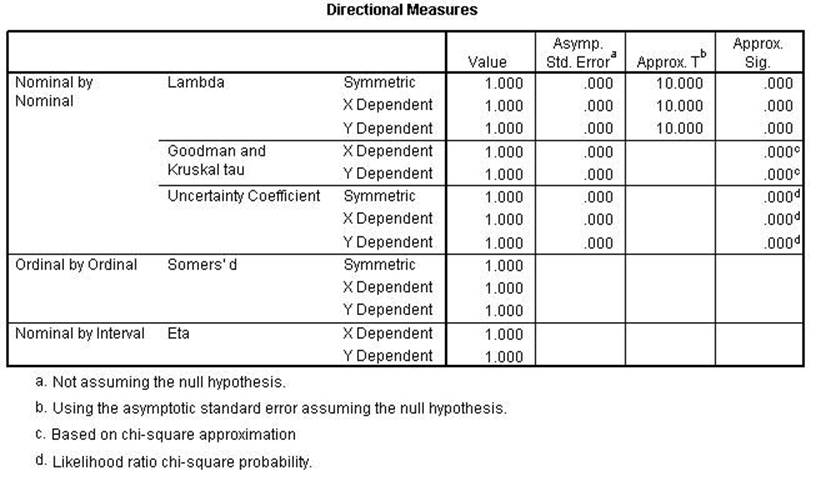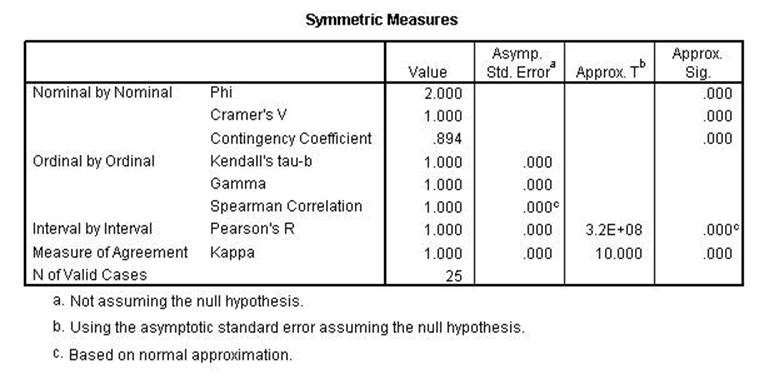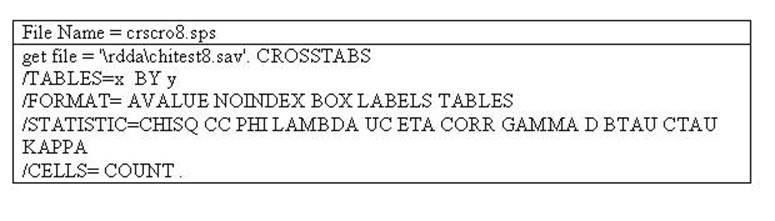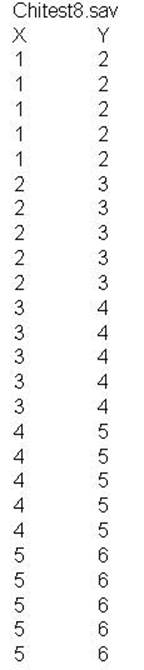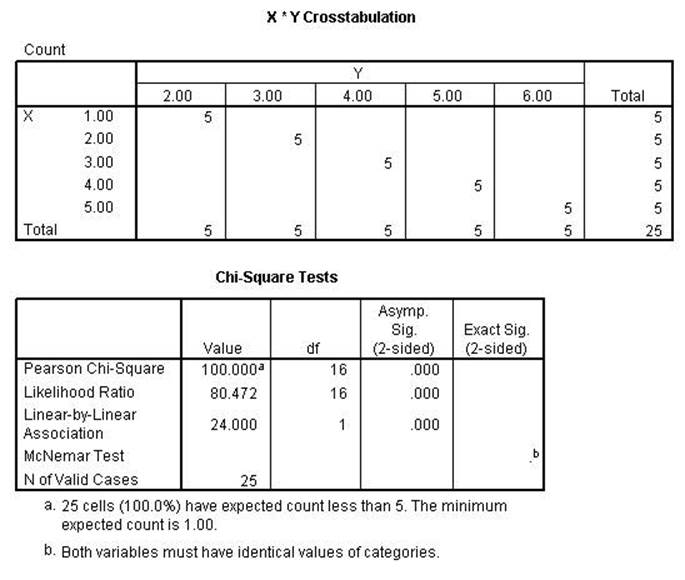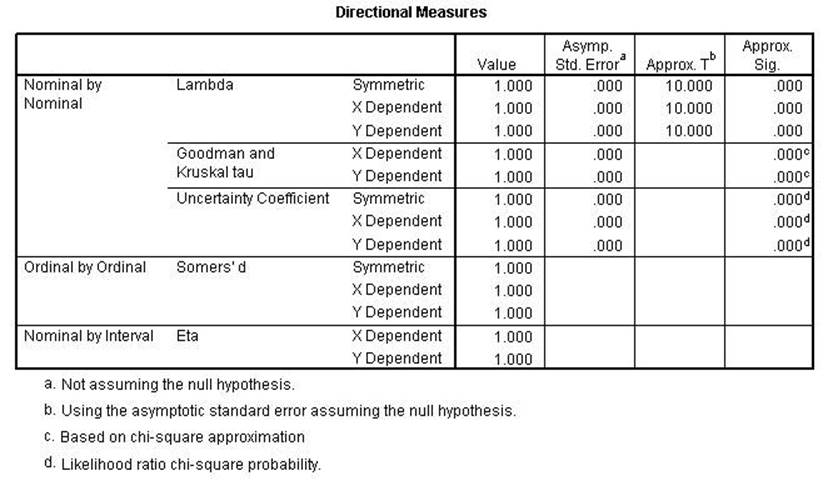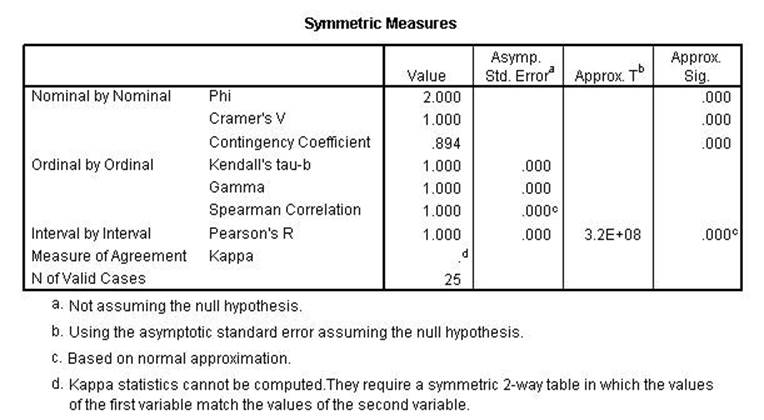The next analysis shows the difference between those statistics that show a linear relationship and those that show a conditional relationship. Those that retain perfect relationships are conditional while those that are less than perfect show linear relationships.

The following syntax file and data produces the resulting output.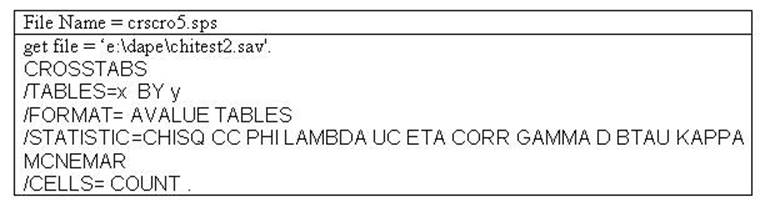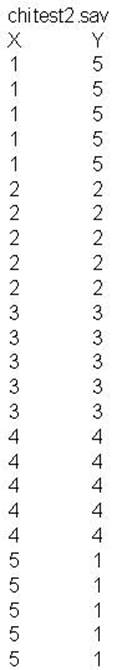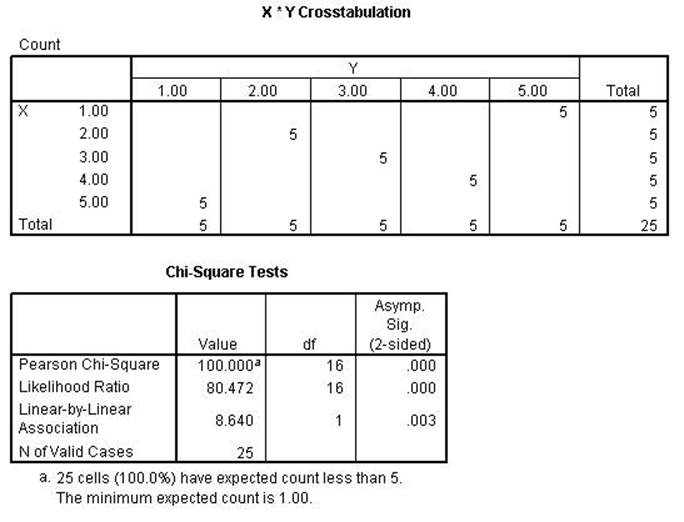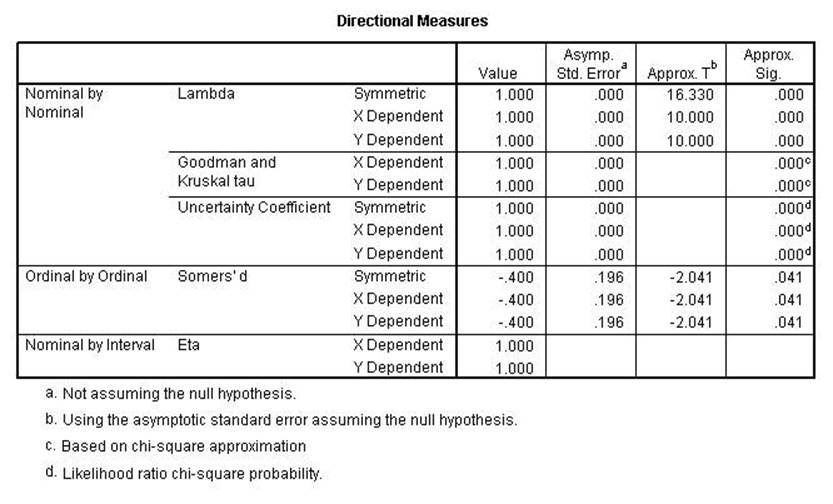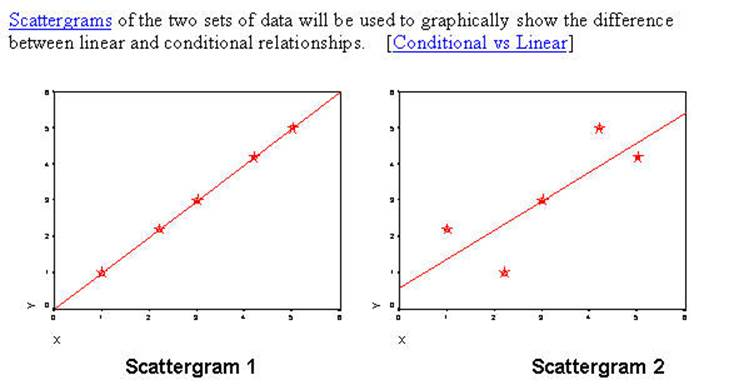In the two scattergrams above there are five cases for each of the circle/stars representing the data from the two examples. Scattergram 1 is generated from the data set CHITEST1 and Scattergram 2 is generated from the data set CHITEST2. For this discussion the Pearson Correlation and the Pearson Chi Square will be the examplars. The table below is a summary of other statistics representing linear or conditional relationships. Those that remain perfect relationships (for the CHITEST2 data) are conditional relationships while those that become less than perfect are linear relationships. The difference between the two can be seen in the two scattergrams. In both situations when you know the value of X then you know the value of Y. In the linear relationship there is an additional requirement that if X is higher on the scale then Y must also be higher on the scale. In Scattergram 2 that is not always the true. Where it is seen that when X is 1 the Y's are all 2's. The Y's are lower. Consequently, the linear relationship does not hold in the second example. However, for conditional relationship where the only requirement is that if you know the value of X then predict that Y is a specific value then those statistics that test such a condition remain perfect. The Pearson Correlation represents the linear relationship and the Pearson Chi Square represents the conditional relationship.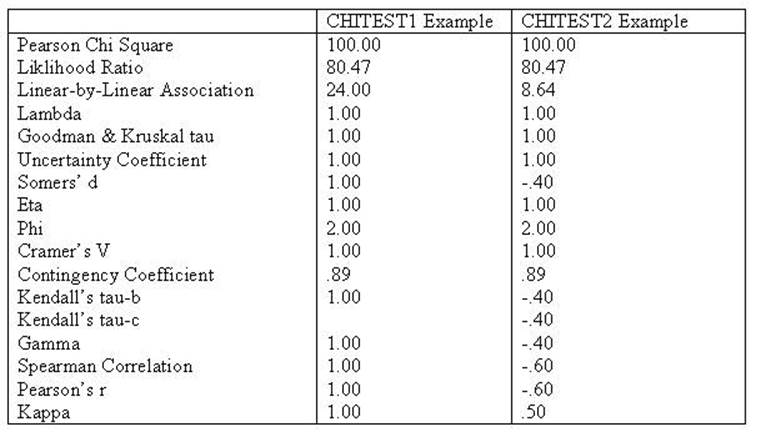Notice that in the left scattergram all points are on the regression line indicating a perfect relationship using the Pearson Correlation Coefficient. In the right scattergram the Pearson will be less than perfect because not all points are on the regression line. The next computer run shows that those statistics that assume linear direction will be lowered while those that do not will remain perfect relationships (conditional probabilities).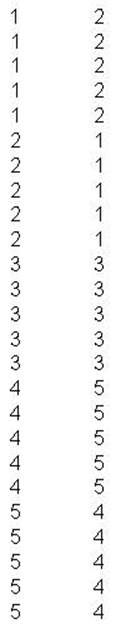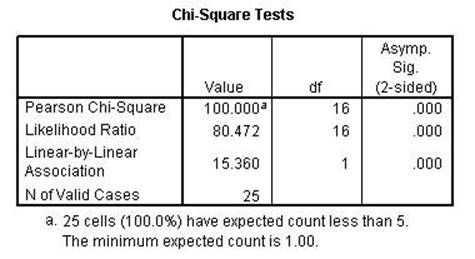The Chi-square and the Likelihood Ratio remained perfect while the Linear-by-Linear Association is lower than the previous run. That indicates that the first two are conditional while the 3rd is linear as the name implies.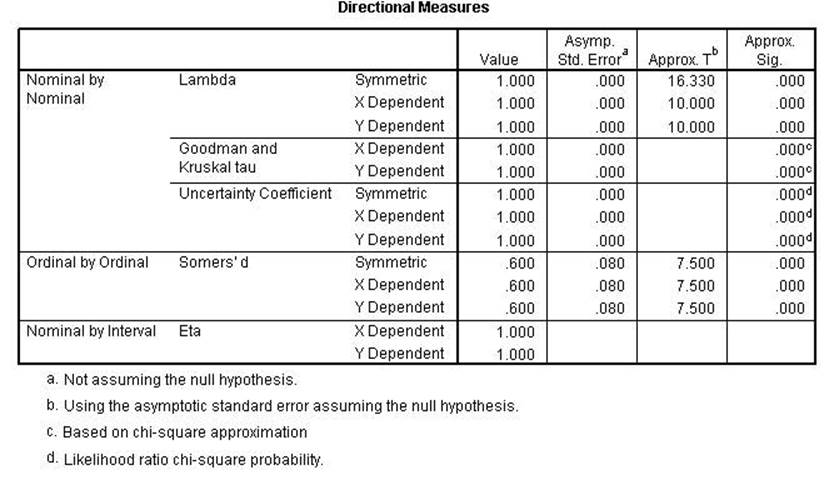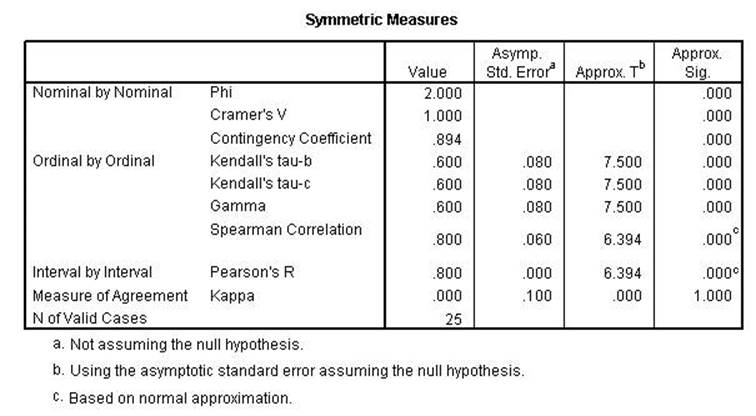Chi Square, Lambda, uncertainty coefficient, Phi, Cramer's V and the Contingency Coefficient all remain the same indicating conditional relationships. Kendall's tau, Gamma, Spearman Correlation, Pearson's r and Kappa were all lower indicating a linear relationshsip.